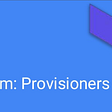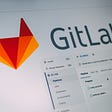# Happy Number Solution in Go Lang

Hey, Glad you found a article that mentions about solving the Happy Number problem in Go lang, because when I started practicing problem solving challenges there we hardly any content around solving programming problems using go lang

So to begin with lets see what actually a Happy number problem is

Happy Number: “A happy number is a number defined by the following process: Starting with any positive integer, replace the number by the sum of the squares of its digits, and repeat the process until the number equals 1 (where it will stay), or it loops endlessly in a cycle which does not include 1. Those numbers for which this process ends in 1 are happy numbers.”

Example:

`Input: 19Output: trueExplanation: 12 + 92 = 8282 + 22 = 6862 + 82 = 10012 + 02 + 02 = 1`

Solution:

The above problem may sound simple or difficult to you, so no matter whichever way you feel about it, the main crux of the problem lies in the highlighted text “loops endlessly in a cycle” of the problem statement.

The idea behind the solution is we know when to stop in case the number is happy i.e “process ends in 1 are happy numbers” but there is no mention of when to stop for a Non-happy input, what is the condition for that, we can’t keep running the process forever and the solution expects the result in form of True or False

So the main Idea is to keep running the program until a loop is not find in the square sequence

Let’s see with an example

`Input: 16Output: falseExplanation: 1 + 36= 379 + 49 = 5825 + 64 = 8964 + 81 = 1451 + 16 + 25 = 4216 + 4 = 204 + 0 = 416 = 161 + 36 = 37 //repeated as line one and going further will be cycle`

If you could notice we started with 16 as input and we got 37 as the first sum of digit squares also at the end of program we again came at 16 causing to create a loop in the process. if we would continue this process we are gonna be stuck in an endless loop between digits from 16 –37–58- …. -4–16 and this is were we realize that we need to stop and the number is not a Happy number resulting in an output as false.

So let’s see the Go lang implementation of solution. We are going to solve this problem in two different ways, one is using map data structure and other one using “Floyd’s cycle finding” Algorithm

## Using Map type

In this variety of solution every time when we calculate a sum of square of digits for a number we add that number into our map, and then we add a check if ever we come across a number that already has a value in map we can conclude that this path is already traveled and we are going to enter into a endless loop and that’s when we break, resulting into a false output for Non-happy number, hope there is no need to mention what if its a happy number

Using “Floyd’s Cycle-Finding ” Algorithm

This is an another way to solve happy number problem which is quite space effective

This is another way to solve happy number problem which is quite space effective

In this approach we run through the numbers at parallel with a speed of, slow:= one jump in square of digits in number

and

fast:= two jumps in square of digits in number

Example

When

Slow= 1 + 36= 37

Fast= (1+36=37)=>(3*3+7*7)=>58

This way we run throughout the loop at different speed and expect to meet at a edge of the loop at one instance which helps us to find the loop and break the chain, if both slow and fast counter met at 1 then it’s a happy number and if its not one they met at a loop and its not a happy number

--

--

--

## More from Go Lang solution for Coding challenges

It’s very hard to find Go lang implementation for Problem solving challenges & algorithms so, here am trying to build it up

## Understanding Stack Overflow Users## Jelastic Named Cloud Service Provider of the Year at Datacloud Awards 2019## TOP 5 PAIN POINTS WHEN DEVELOPING NEW SOFTWARE## A Simple Way to Manage Data Science Assets with IBM Watson Machine Learning API## This IDE Will Be Important## Terraform remote-exec provisioner with Azure## Sunil Suseelan

Let me Brag about myself, something that no one does on social platform ;). Well to be frank am not a super technical guy, but I strive to do my best

## Concurrency in Go ( Beginners )## Learning Go: Introduction## 3 ways to tackle the longest increasing subsequence problem(Golang)